Related Articles
Playfair Cipher with Examples
• Difficulty Level : Easy
• Last Updated : 06 May, 2021

The Playfair cipher was the first practical digraph substitution cipher. The scheme was invented in 1854 by Charles Wheatstone but was named after Lord Playfair who promoted the use of the cipher. In playfair cipher unlike traditional cipher we encrypt a pair of alphabets(digraphs) instead of a single alphabet.
It was used for tactical purposes by British forces in the Second Boer War and in World War I and for the same purpose by the Australians during World War II. This was because Playfair is reasonably fast to use and requires no special equipment.

Encryption Technique

For the encryption process let us consider the following example:The Playfair Cipher Encryption Algorithm:
The Algorithm consistes of 2 steps:

1. Generate the key Square(5×5):
• The key square is a 5×5 grid of alphabets that acts as the key for encrypting the plaintext. Each of the 25 alphabets must be unique and one letter of the alphabet (usually J) is omitted from the table (as the table can hold only 25 alphabets). If the plaintext contains J, then it is replaced by I.

• The initial alphabets in the key square are the unique alphabets of the key in the order in which they appear followed by the remaining letters of the alphabet in order.

2. Algorithm to encrypt the plain text: The plaintext is split into pairs of two letters (digraphs). If there is an odd number of letters, a Z is added to the last letter.
For example:

```PlainText: "instruments"
After Split: 'in' 'st' 'ru' 'me' 'nt' 'sz'```

1. Pair cannot be made with same letter. Break the letter in single and add a bogus letter to the previous letter.

Plain Text: “hello”

After Split: ‘he’ ‘lx’ ‘lo’

Here ‘x’ is the bogus letter.

2. If the letter is standing alone in the process of pairing, then add an extra bogus letter with the alone letter

Plain Text: “helloe”

AfterSplit: ‘he’ ‘lx’ ‘lo’ ‘lz’

Here ‘z’  is the bogus letter.

Rules for Encryption:

• If both the letters are in the same column: Take the letter below each one (going back to the top if at the bottom).
For example:

```Diagraph: "me"
Encrypted Text: cl
Encryption:
m -> c
e -> l```
•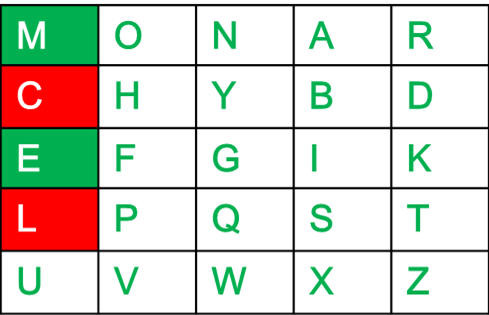•
• If both the letters are in the same row: Take the letter to the right of each one (going back to the leftmost if at the rightmost position).
For example:

```Diagraph: "st"
Encrypted Text: tl
Encryption:
s -> t
t -> l```
•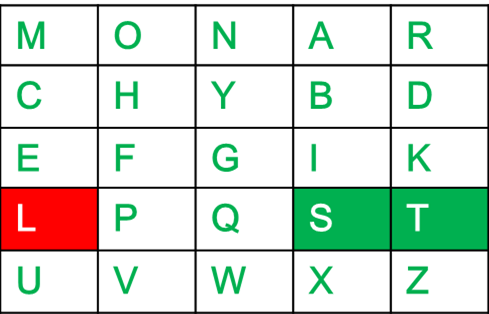•
• If neither of the above rules is true: Form a rectangle with the two letters and take the letters on the horizontal opposite corner of the rectangle.
For example:

```Diagraph: "nt"
Encrypted Text: rq
Encryption:
n -> r
t -> q```
•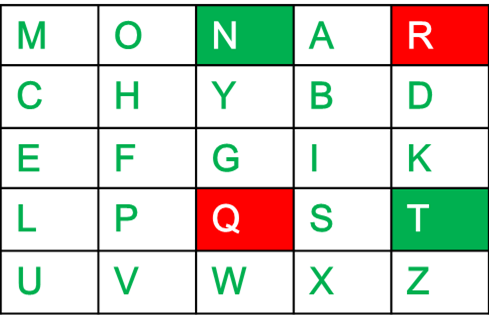•

For example:

```Plain Text: "instrumentsz"
Encrypted Text: gatlmzclrqtx
Encryption:
i -> g
n -> a
s -> t
t -> l
r -> m
u -> z
m -> c
e -> l
n -> r
t -> q
s -> t
z -> x```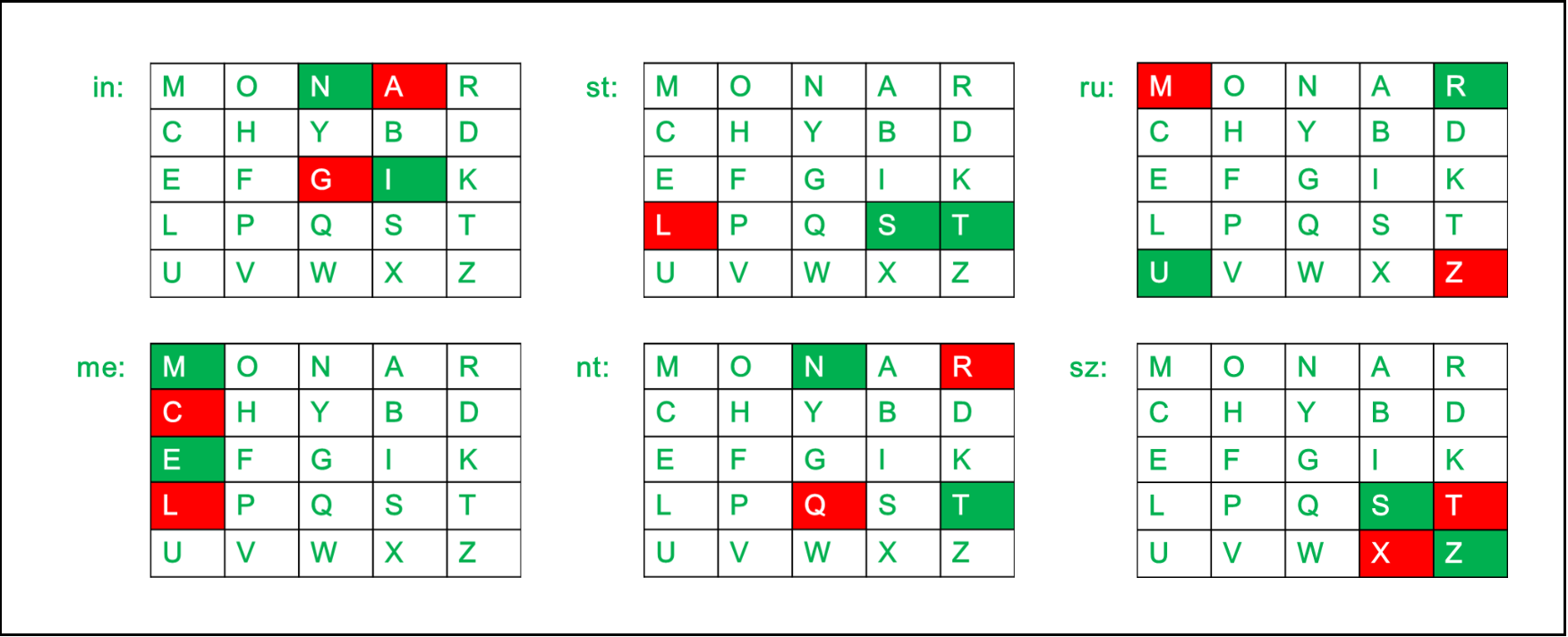Below is an implementation of Playfair Cipher in C:

## C

 `// C program to implement Playfair Cipher` `#include ``#include ``#include ` `#define SIZE 30` `// Function to convert the string to lowercase``void` `toLowerCase(``char` `plain[], ``int` `ps)``{``    ``int` `i;``    ``for` `(i = 0; i < ps; i++) {``        ``if` `(plain[i] > 64 && plain[i] < 91)``            ``plain[i] += 32;``    ``}``}` `// Function to remove all spaces in a string``int` `removeSpaces(``char``* plain, ``int` `ps)``{``    ``int` `i, count = 0;``    ``for` `(i = 0; i < ps; i++)``        ``if` `(plain[i] != ``' '``)``            ``plain[count++] = plain[i];``    ``plain[count] = ``'\0'``;``    ``return` `count;``}` `// Function to generate the 5x5 key square``void` `generateKeyTable(``char` `key[], ``int` `ks, ``char` `keyT)``{``    ``int` `i, j, k, flag = 0, *dicty;` `    ``// a 26 character hashmap``    ``// to store count of the alphabet``    ``dicty = (``int``*)``calloc``(26, ``sizeof``(``int``));``    ``for` `(i = 0; i < ks; i++) {``        ``if` `(key[i] != ``'j'``)``            ``dicty[key[i] - 97] = 2;``    ``}` `    ``dicty[``'j'` `- 97] = 1;` `    ``i = 0;``    ``j = 0;` `    ``for` `(k = 0; k < ks; k++) {``        ``if` `(dicty[key[k] - 97] == 2) {``            ``dicty[key[k] - 97] -= 1;``            ``keyT[i][j] = key[k];``            ``j++;``            ``if` `(j == 5) {``                ``i++;``                ``j = 0;``            ``}``        ``}``    ``}` `    ``for` `(k = 0; k < 26; k++) {``        ``if` `(dicty[k] == 0) {``            ``keyT[i][j] = (``char``)(k + 97);``            ``j++;``            ``if` `(j == 5) {``                ``i++;``                ``j = 0;``            ``}``        ``}``    ``}``}` `// Function to search for the characters of a digraph``// in the key square and return their position``void` `search(``char` `keyT, ``char` `a, ``char` `b, ``int` `arr[])``{``    ``int` `i, j;` `    ``if` `(a == ``'j'``)``        ``a = ``'i'``;``    ``else` `if` `(b == ``'j'``)``        ``b = ``'i'``;` `    ``for` `(i = 0; i < 5; i++) {` `        ``for` `(j = 0; j < 5; j++) {` `            ``if` `(keyT[i][j] == a) {``                ``arr = i;``                ``arr = j;``            ``}``            ``else` `if` `(keyT[i][j] == b) {``                ``arr = i;``                ``arr = j;``            ``}``        ``}``    ``}``}` `// Function to find the modulus with 5``int` `mod5(``int` `a)``{``    ``return` `(a % 5);``}` `// Function to make the plain text length to be even``int` `prepare(``char` `str[], ``int` `ptrs)``{``    ``if` `(ptrs % 2 != 0) {``        ``str[ptrs++] = ``'z'``;``        ``str[ptrs] = ``'\0'``;``    ``}``    ``return` `ptrs;``}` `// Function for performing the encryption``void` `encrypt(``char` `str[], ``char` `keyT, ``int` `ps)``{``    ``int` `i, a;` `    ``for` `(i = 0; i < ps; i += 2) {` `        ``search(keyT, str[i], str[i + 1], a);` `        ``if` `(a == a) {``            ``str[i] = keyT[a][mod5(a + 1)];``            ``str[i + 1] = keyT[a][mod5(a + 1)];``        ``}``        ``else` `if` `(a == a) {``            ``str[i] = keyT[mod5(a + 1)][a];``            ``str[i + 1] = keyT[mod5(a + 1)][a];``        ``}``        ``else` `{``            ``str[i] = keyT[a][a];``            ``str[i + 1] = keyT[a][a];``        ``}``    ``}``}` `// Function to encrypt using Playfair Cipher``void` `encryptByPlayfairCipher(``char` `str[], ``char` `key[])``{``    ``char` `ps, ks, keyT;` `    ``// Key``    ``ks = ``strlen``(key);``    ``ks = removeSpaces(key, ks);``    ``toLowerCase(key, ks);` `    ``// Plaintext``    ``ps = ``strlen``(str);``    ``toLowerCase(str, ps);``    ``ps = removeSpaces(str, ps);` `    ``ps = prepare(str, ps);` `    ``generateKeyTable(key, ks, keyT);` `    ``encrypt(str, keyT, ps);``}` `// Driver code``int` `main()``{``    ``char` `str[SIZE], key[SIZE];` `    ``// Key to be encrypted``    ``strcpy``(key, ``"Monarchy"``);``    ``printf``(``"Key text: %s\n"``, key);` `    ``// Plaintext to be encrypted``    ``strcpy``(str, ``"instruments"``);``    ``printf``(``"Plain text: %s\n"``, str);` `    ``// encrypt using Playfair Cipher``    ``encryptByPlayfairCipher(str, key);` `    ``printf``(``"Cipher text: %s\n"``, str);` `    ``return` `0;``}` `// This code is contributed by AbhayBhat`
Output:
```Key text: Monarchy
Plain text: instruments
Cipher text: gatlmzclrqtx```

Decryption Technique

Decrypting the Playfair cipher is as simple as doing the same process in reverse. The receiver has the same key and can create the same key table, and then decrypt any messages made using that key.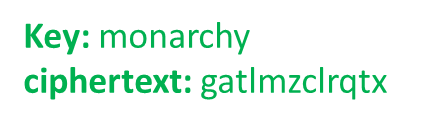The Playfair Cipher Decryption Algorithm:
The Algorithm consistes of 2 steps:

1. Generate the key Square(5×5) at the receiver’s end:
• The key square is a 5×5 grid of alphabets that acts as the key for encrypting the plaintext. Each of the 25 alphabets must be unique and one letter of the alphabet (usually J) is omitted from the table (as the table can hold only 25 alphabets). If the plaintext contains J, then it is replaced by I.

• The initial alphabets in the key square are the unique alphabets of the key in the order in which they appear followed by the remaining letters of the alphabet in order.

2. Algorithm to decrypt the ciphertext: The ciphertext is split into pairs of two letters (digraphs).

Note: The ciphertext always have even number of characters.

1. For example:

```CipherText: "gatlmzclrqtx"
After Split: 'ga' 'tl' 'mz' 'cl' 'rq' 'tx'```
1. Rules for Decryption:
• If both the letters are in the same column: Take the letter above each one (going back to the bottom if at the top).
For example:

```Diagraph: "cl"
Decrypted Text: me
Decryption:
c -> m
l -> e```
••
• If both the letters are in the same row: Take the letter to the left of each one (going back to the rightmost if at the leftmost position).
For example:

```Diagraph: "tl"
Decrypted Text: st
Decryption:
t -> s
l -> t```
••
• If neither of the above rules is true: Form a rectangle with the two letters and take the letters on the horizontal opposite corner of the rectangle.
For example:

```Diagraph: "rq"
Decrypted Text: nt
Decryption:
r -> n
q -> t```
••

For example:

```Plain Text: "gatlmzclrqtx"
Decrypted Text: instrumentsz
Decryption:
(red)-> (green)
ga -> in
tl -> st
mz -> ru
cl -> me
rq -> nt
tx -> sz```Below is an implementation of Playfair Cipher Decryption in C:

## C

 `#include ``#include ``#include ``#define SIZE 30` `// Convert all the characters``// of a string to lowercase``void` `toLowerCase(``char` `plain[], ``int` `ps)``{``    ``int` `i;``    ``for` `(i = 0; i < ps; i++) {``        ``if` `(plain[i] > 64 && plain[i] < 91)``            ``plain[i] += 32;``    ``}``}` `// Remove all spaces in a string``// can be extended to remove punctuation``int` `removeSpaces(``char``* plain, ``int` `ps)``{``    ``int` `i, count = 0;``    ``for` `(i = 0; i < ps; i++)``        ``if` `(plain[i] != ``' '``)``            ``plain[count++] = plain[i];``    ``plain[count] = ``'\0'``;``    ``return` `count;``}` `// generates the 5x5 key square``void` `generateKeyTable(``char` `key[], ``int` `ks,``                      ``char` `keyT)``{``    ``int` `i, j, k, flag = 0, *dicty;` `    ``// a 26 character hashmap``    ``// to store count of the alphabet``    ``dicty = (``int``*)``calloc``(26, ``sizeof``(``int``));` `    ``for` `(i = 0; i < ks; i++) {``        ``if` `(key[i] != ``'j'``)``            ``dicty[key[i] - 97] = 2;``    ``}``    ``dicty[``'j'` `- 97] = 1;` `    ``i = 0;``    ``j = 0;``    ``for` `(k = 0; k < ks; k++) {``        ``if` `(dicty[key[k] - 97] == 2) {``            ``dicty[key[k] - 97] -= 1;``            ``keyT[i][j] = key[k];``            ``j++;``            ``if` `(j == 5) {``                ``i++;``                ``j = 0;``            ``}``        ``}``    ``}``    ``for` `(k = 0; k < 26; k++) {``        ``if` `(dicty[k] == 0) {``            ``keyT[i][j] = (``char``)(k + 97);``            ``j++;``            ``if` `(j == 5) {``                ``i++;``                ``j = 0;``            ``}``        ``}``    ``}``}` `// Search for the characters of a digraph``// in the key square and return their position``void` `search(``char` `keyT, ``char` `a,``            ``char` `b, ``int` `arr[])``{``    ``int` `i, j;` `    ``if` `(a == ``'j'``)``        ``a = ``'i'``;``    ``else` `if` `(b == ``'j'``)``        ``b = ``'i'``;` `    ``for` `(i = 0; i < 5; i++) {``        ``for` `(j = 0; j < 5; j++) {``            ``if` `(keyT[i][j] == a) {``                ``arr = i;``                ``arr = j;``            ``}``            ``else` `if` `(keyT[i][j] == b) {``                ``arr = i;``                ``arr = j;``            ``}``        ``}``    ``}``}` `// Function to find the modulus with 5``int` `mod5(``int` `a)``{``    ``return` `(a % 5);``}` `// Function to decrypt``void` `decrypt(``char` `str[], ``char` `keyT, ``int` `ps)``{``    ``int` `i, a;``    ``for` `(i = 0; i < ps; i += 2) {``        ``search(keyT, str[i], str[i + 1], a);``        ``if` `(a == a) {``            ``str[i] = keyT[a][mod5(a - 1)];``            ``str[i + 1] = keyT[a][mod5(a - 1)];``        ``}``        ``else` `if` `(a == a) {``            ``str[i] = keyT[mod5(a - 1)][a];``            ``str[i + 1] = keyT[mod5(a - 1)][a];``        ``}``        ``else` `{``            ``str[i] = keyT[a][a];``            ``str[i + 1] = keyT[a][a];``        ``}``    ``}``}` `// Function to call decrypt``void` `decryptByPlayfairCipher(``char` `str[], ``char` `key[])``{``    ``char` `ps, ks, keyT;` `    ``// Key``    ``ks = ``strlen``(key);``    ``ks = removeSpaces(key, ks);``    ``toLowerCase(key, ks);` `    ``// ciphertext``    ``ps = ``strlen``(str);``    ``toLowerCase(str, ps);``    ``ps = removeSpaces(str, ps);` `    ``generateKeyTable(key, ks, keyT);` `    ``decrypt(str, keyT, ps);``}` `// Driver code``int` `main()``{``    ``char` `str[SIZE], key[SIZE];` `    ``// Key to be encrypted``    ``strcpy``(key, ``"Monarchy"``);``    ``printf``(``"Key text: %s\n"``, key);` `    ``// Ciphertext to be decrypted``    ``strcpy``(str, ``"gatlmzclrqtx"``);``    ``printf``(``"Plain text: %s\n"``, str);` `    ``// encrypt using Playfair Cipher``    ``decryptByPlayfairCipher(str, key);` `    ``printf``(``"Deciphered text: %s\n"``, str);` `    ``return` `0;``}` `// This code is contributed by AbhayBhat`
Output:
```Key text: Monarchy
Plain text: gatlmzclrqtx
Deciphered text: inskrumentsz```

1. It is significantly harder to break since the frequency analysis technique used to break simple substitution ciphers is difficult but still can be used on (25*25) = 625 digraphs rather than 25 monographs which is difficult.

2. Frequency analysis thus requires more cipher text to crack the encryption.